# CBSE Class 12 Physics Chapter 8 Revision Notes

Chapter 8: Electromagnetic Waves Revision Notes

• Displacement Current: The current that is generated in the region where the electric field and electric flux are changing over time.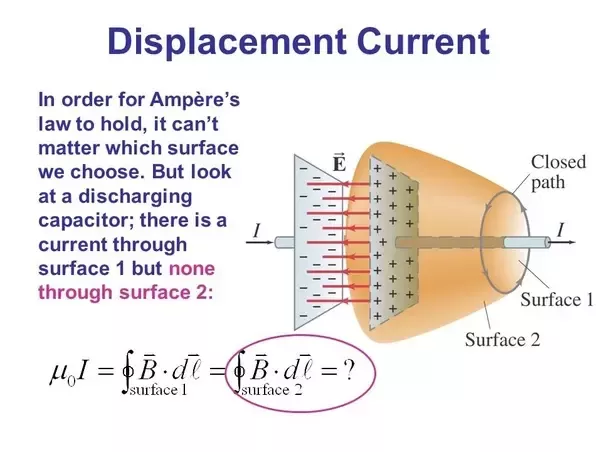Source

• Need for Displacement Current: The circuital law of Ampere for conduction current during capacitor charging was found to be inconclusive. As a result, Maxwell made changes to Ampere’s circuital law.
• A displacement current is created in space as a result of a change in electric flux at the surface. This reveals that the source of the magnetic field is a changing electric field.

## Maxwell’s Electromagnetic Wave Equations

• The basic laws of electricity and magnetism are Maxwell’s equations. These equations describe all electromagnetic interactions in detail.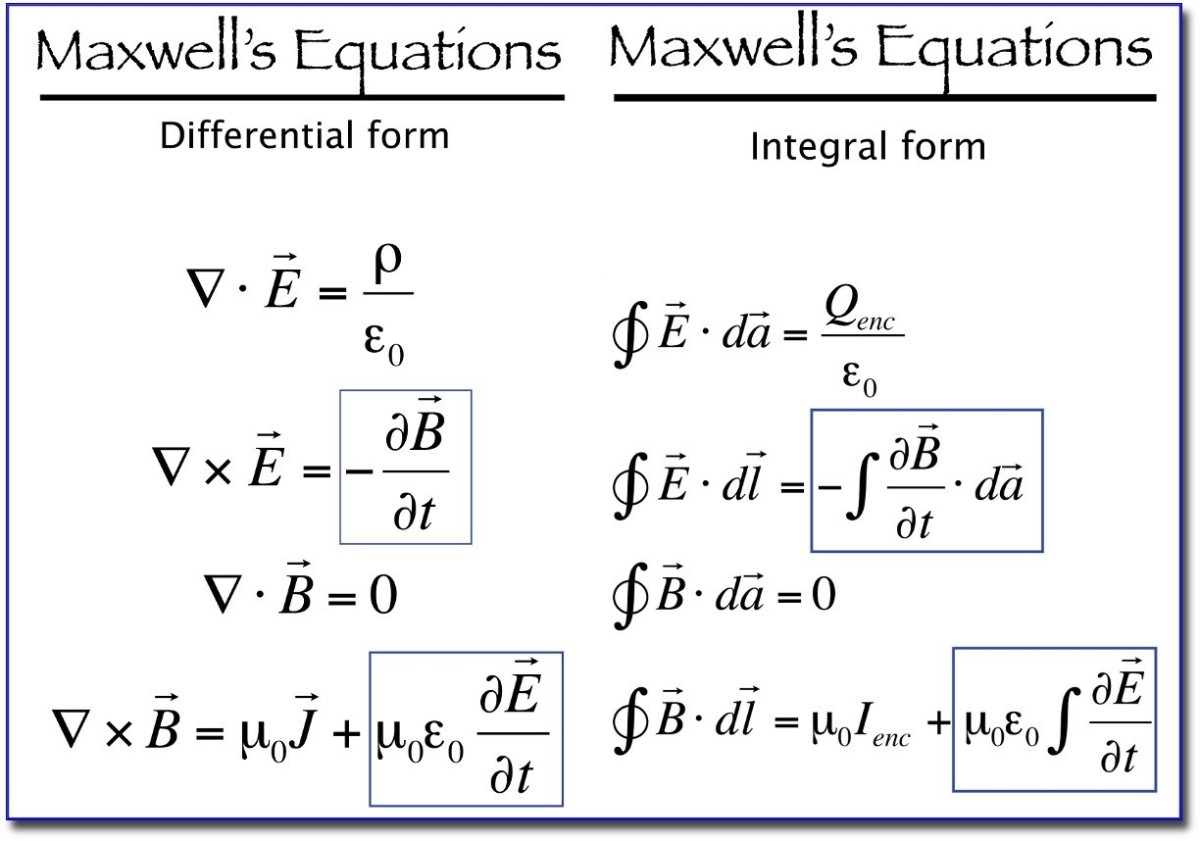Source

• Electromagnetic Waves: An electromagnetic wave is a wave emitted by an accelerated or oscillating charge in which the electric field is generated by a varying magnetic field and the magnetic field is generated by a varying electric field.
• As a result, two fields become sources for each other, and the wave propagates in a perpendicular direction to both fields.
• Electromagnetic waves are transverse in nature, which means that the electric and magnetic fields are perpendicular to each other and the wave propagation direction. Electric and magnetic fields do not deflect electromagnetic waves.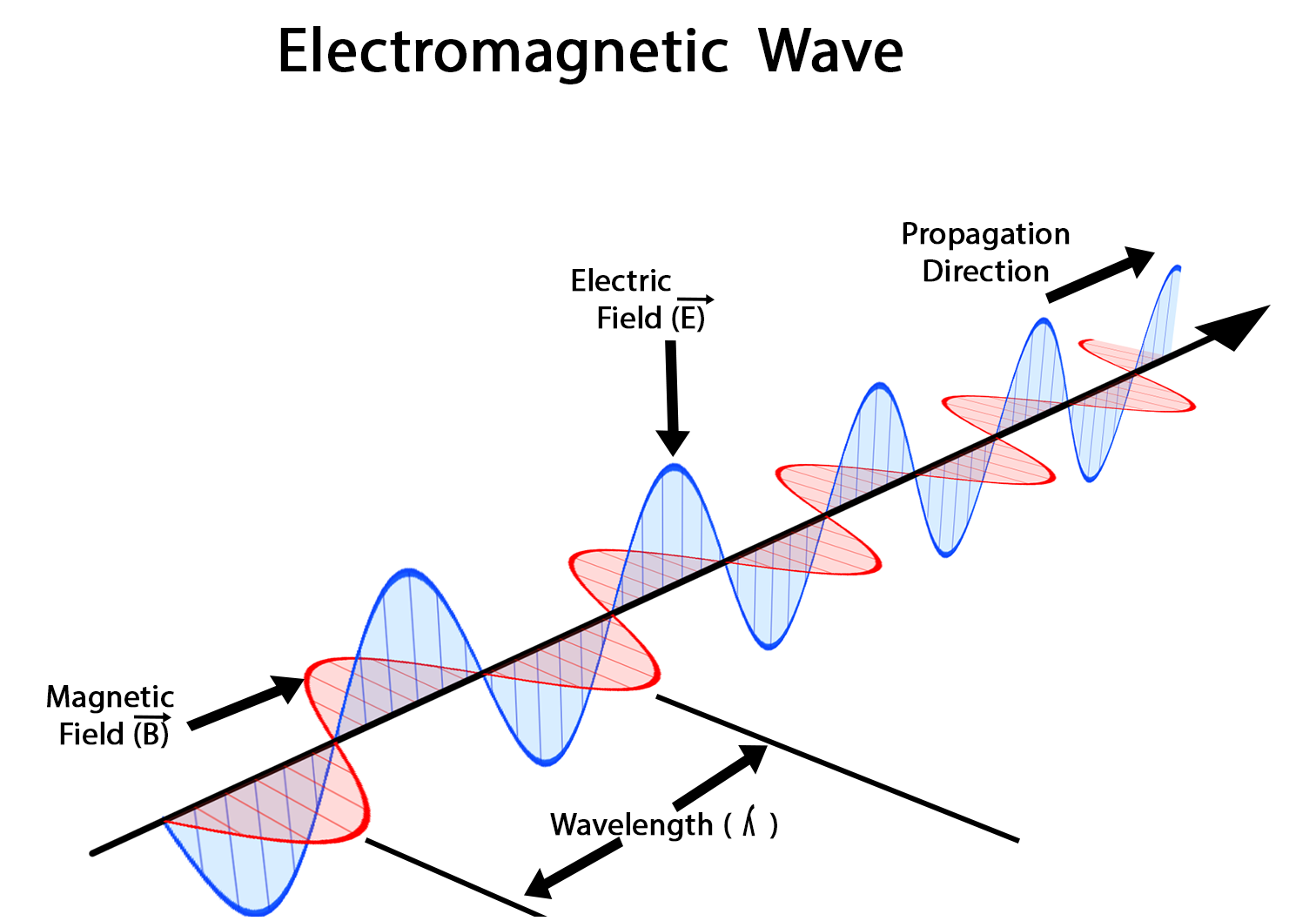Source

• Electromagnetic waves have the same phase of E (electric field) and B (magnetic field).

## Electromagnetic wave speed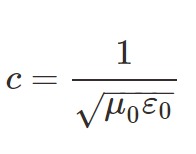Source

Here, ε0 is the permittivity of free space and μ0 is the permeability of free space (vacuum) and is a physical constant equal to 1.257×10−6H/m(approximately).

• The energy in an electromagnetic wave is split evenly between electric and magnetic fields on average.

• Linear momentum delivered to the surface is given by,

p= U/c,

where U represents the total energy transmitted by electromagnetic waves and c represents the speed of the electromagnetic wave.

## Electromagnetic Spectrum

• It is a diagram that depicts the electromagnetic spectrum. The electromagnetic spectrum is the systematic sequential distribution of electromagnetic waves in ascending or descending order of frequency or wavelength.
• From -rays to radio waves, the range varies from 10-12 m to 104 m.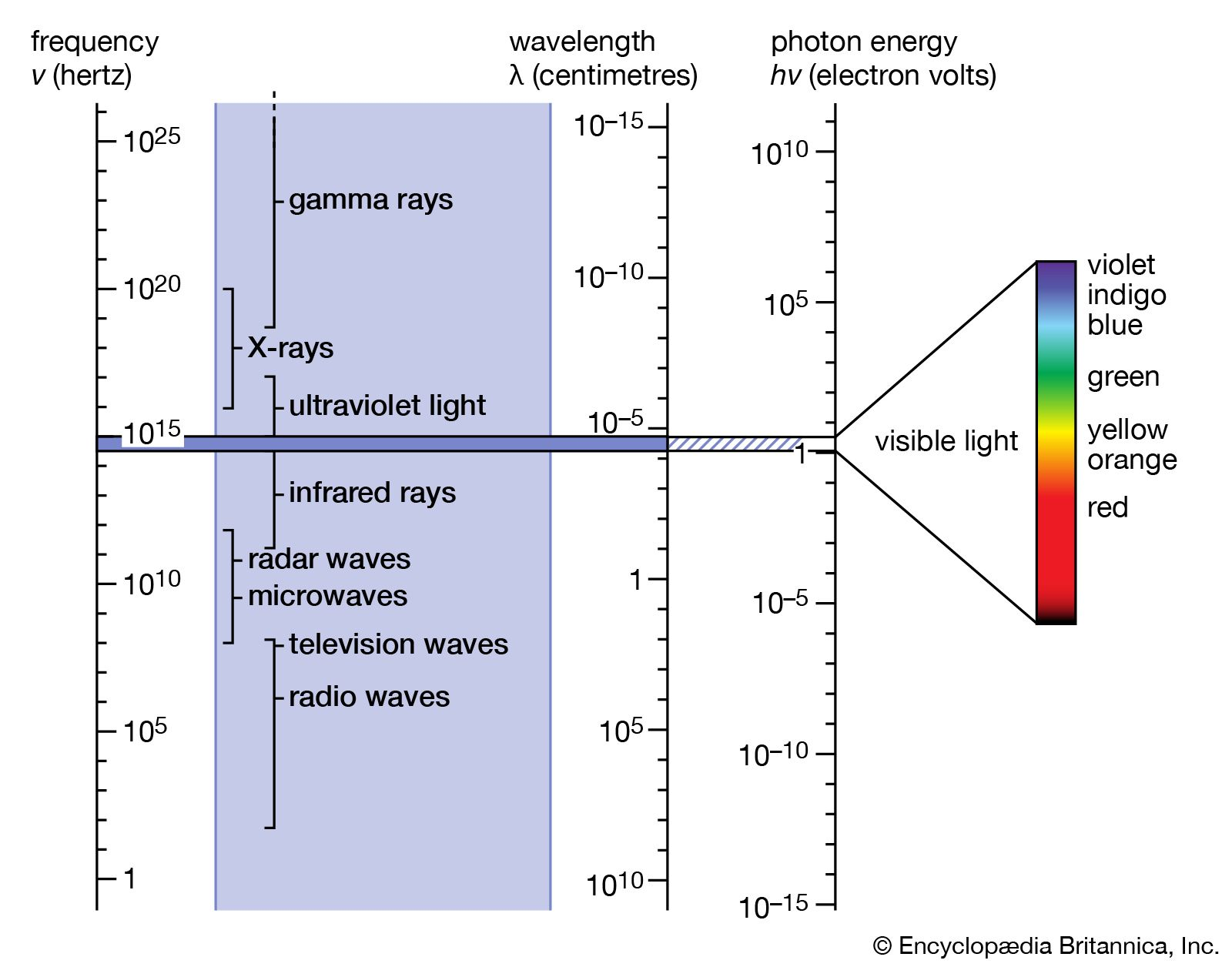Source

• Radio waves: are used in

(ii) In the field of astronomy.

• Microwaves are used in

(ii) In molecular and atomic structure analysis.

(iii) For the purpose of cooking.

• Infrared waves are useful for

(i) Determining molecular structure.

(ii) In TV, VCR, and other remote controls

• UV rays are used in

(i) Burglar alarms.

(ii) To eliminate germs from minerals.

• X-rays are used in

(i) Medical diagnosis because they pass through muscles rather than bones.

(ii) detecting faults, cracks, and other defects in metal products.

• γ-rays are used in

(i) Food preservation

(ii) In the field of radiotherapy.

]]>